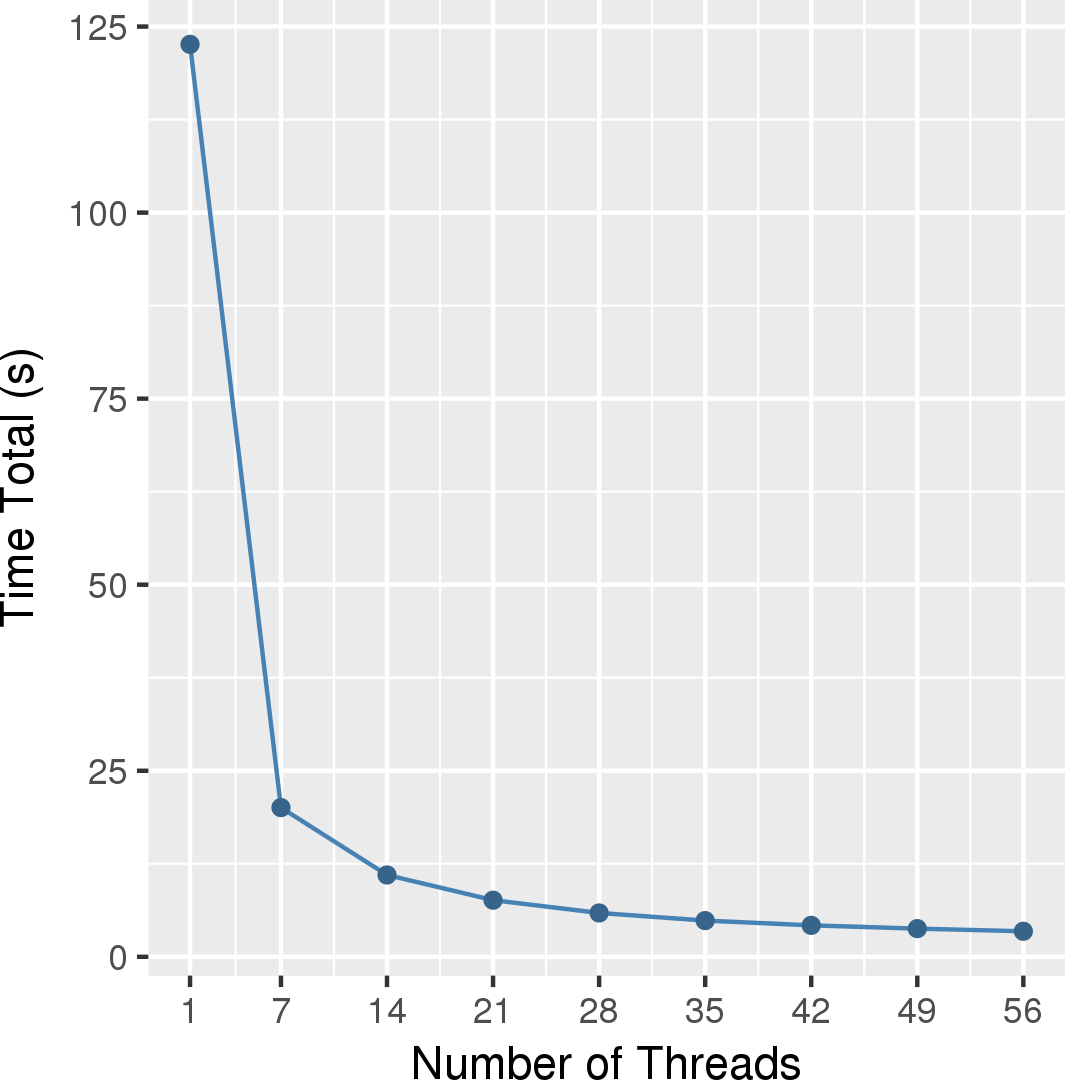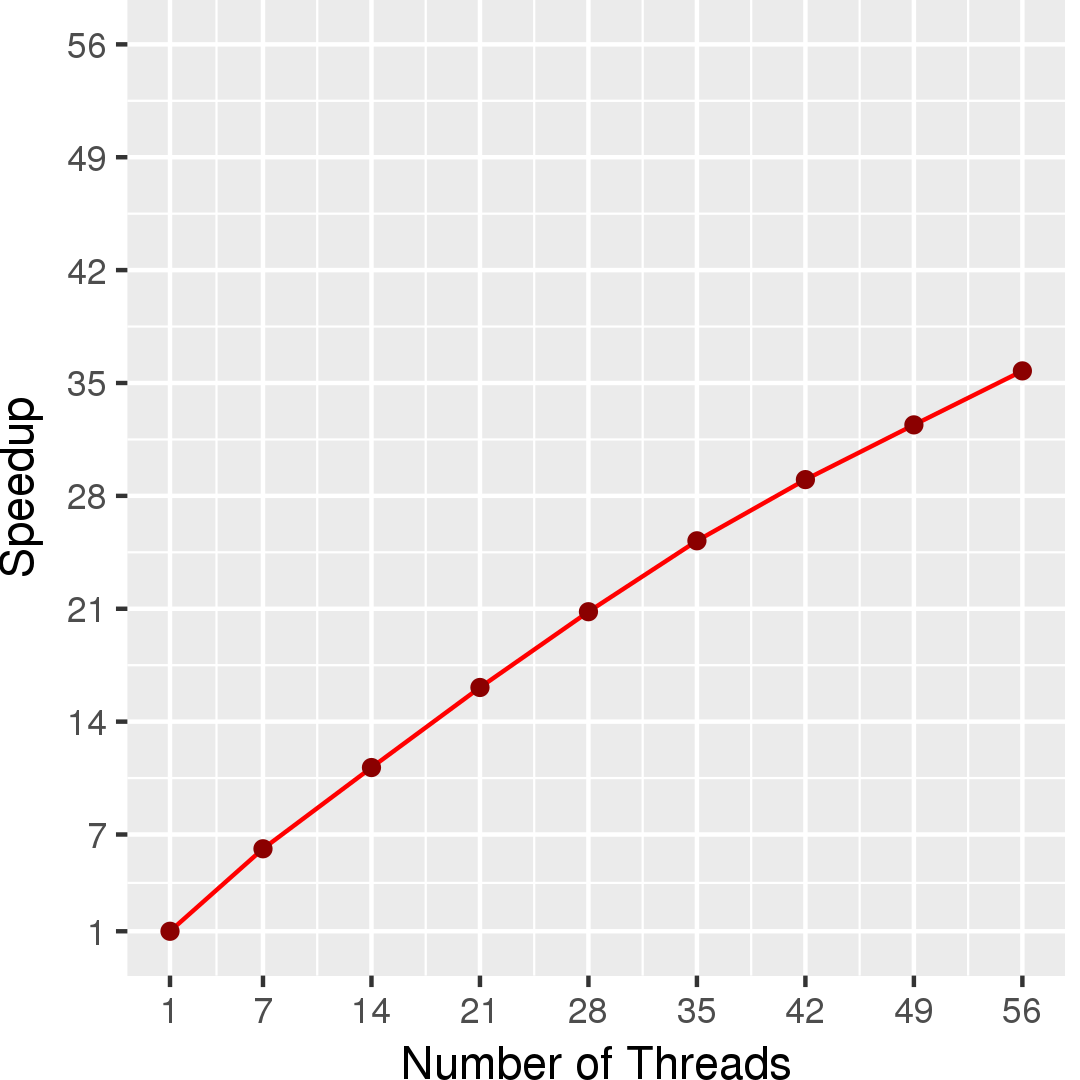# Points-To Analysis

## Application Description

This benchmark takes a set of constraints on a set of variables and determines which variables can point to which other variables given these set of constraints. This is useful for compiler analysis of programs to determine what kind of optimizations can be applied.

There are 4 main constraints that are generally dealt with given a variable $$x$$ and a variable $$y$$ (and that we concern ourselves with in our implementation):

$$x$$ points to $$y$$
Copy
$$x$$ is a copy of $$y$$ (anything that $$y$$ points to $$x$$ points to)
A load from $$x$$ to $$y$$ implies anything $$x$$ points to must also points to $$y$$
Store
A store from $$x$$ to $$y$$ implies $$x$$ must point to anything that $$y$$ points to

## Algorithm

Our implementation is a parallel version of Hardekopf and Lin's serial points-to analysis algorithm  which has been used in gcc and LLVM. We construct points-to analysis as a graph problem: variables are nodes, and copy relations are represented with edges. The initial graph is constructed by processing address of constraints and copy constraints. This determines the initial points-to relations as well as copy relations. All nodes with edges are then added to a worklist.

Threads will individually take nodes from the worklist and propagate points to information along the edges constructed by the copy relationships. Load/store constraints are all periodically handled at certain intervals or when the worklist becomes empty. These constraints may add more edges into the graph: nodes with newly added edges are added to the worklist where points-to propagation will continue along these new edges. Execution continues until there are no more items in the worklist (i.e. no new points-to relationships are made).

This process is represented at a high-level in the psuedo-code below:

 1 2 3 4 5 6 7 8 9 10 11 12 13 Graph g, PointsToRelations p Read constraints Process Address Of and Copy constraints, updating g and p Worklist wl = new Worklist(nodes with edges in g) while (wl is not empty) { foreach (Node a : wl) { propagate a's points-to relations along its edges update p } Process Load and Store constraints, updating g Add nodes with new edges onto worklist }

Figure 1: Pseudocode for Parallel Points-To Analysis.

Our implementation also contains online cycle detection. The program will periodically check the current points-to graph for cycles and collapse the cycles into a single node: this increases efficiency overall as less nodes need to be considered during the algorithm. Currently, only the serial version of our implementation supports online cycle detection.

One of the keys to our efficiency is the use of concurrent sparse bit vectors to represent both the graph and the points-to set. Edges between nodes are represented by bits in a sparse bit vector. The same is true for points-to relationships between nodes.

For a more general overview of the algorithm with more details, see our OOPSLA'10 paper .

## Data Structures

The implementation uses the following key data structures:
Sparse Bit Vector
A sparse bit vector is used to represent both the graph structure and the points-to sets for each node. They are very space efficient since they only grow as you set more bits. Additionally, our implementation is thread-safe: it has enough guarantees that allow points-to analysis to run correctly.

## Performance

Figure 2 and Figure 3 show the execution time in seconds and self-relative speedup (speedup with respect to the single thread performance), respectively, of this algorithm using gdb as the input.B. Hardekopf and C. Lin. The ant and the grasshopper: fast and accurate pointer analysis for millions of lines of code, PLDI 2007.

 M. Mendez-Lojo, A. Matthew, and K. Pingali. Parallel Inclusion-based Points-to Analysis, OOPSLA 2010.

## Machine Description

Performance numbers are collected on a 4 package (14 cores per package) Intel(R) Xeon(R) Gold 5120 CPU machine at 2.20GHz from 1 thread to 56 threads in increments of 7 threads. The machine has 192GB of RAM. The operating system is CentOS Linux release 7.5.1804. All runs of the Galois benchmarks used gcc/g++ 7.2 to compile.